# DPLLbased Checkers for Satisfiability Modulo Theories Cesare Tinelli

• Slides: 40
Download presentation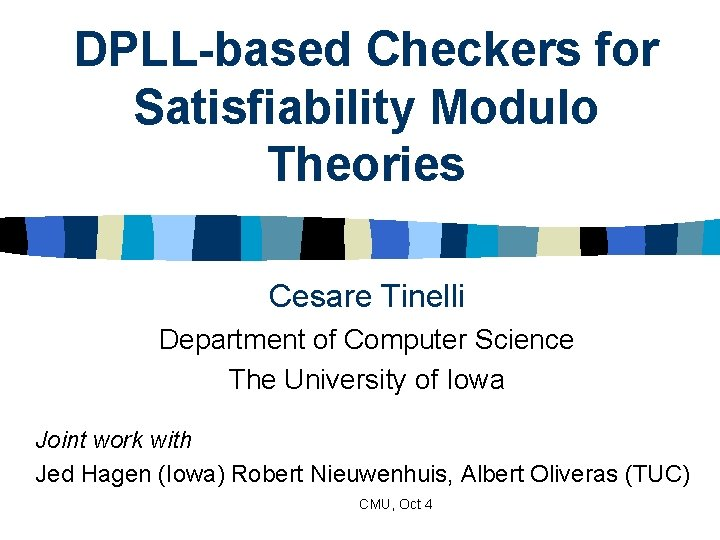DPLL-based Checkers for Satisfiability Modulo Theories Cesare Tinelli Department of Computer Science The University of Iowa Joint work with Jed Hagen (Iowa) Robert Nieuwenhuis, Albert Oliveras (TUC) CMU, Oct 4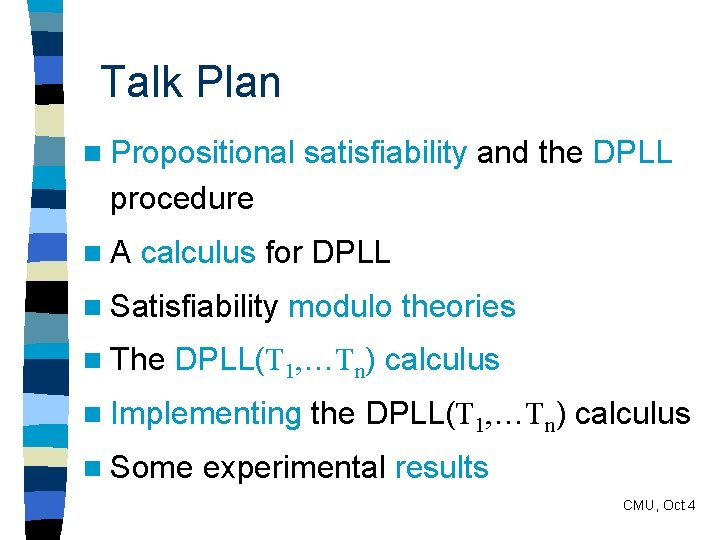Talk Plan n Propositional satisfiability and the DPLL procedure n. A calculus for DPLL n Satisfiability n The modulo theories DPLL(T 1, …Tn) calculus n Implementing n Some the DPLL(T 1, …Tn) calculus experimental results CMU, Oct 4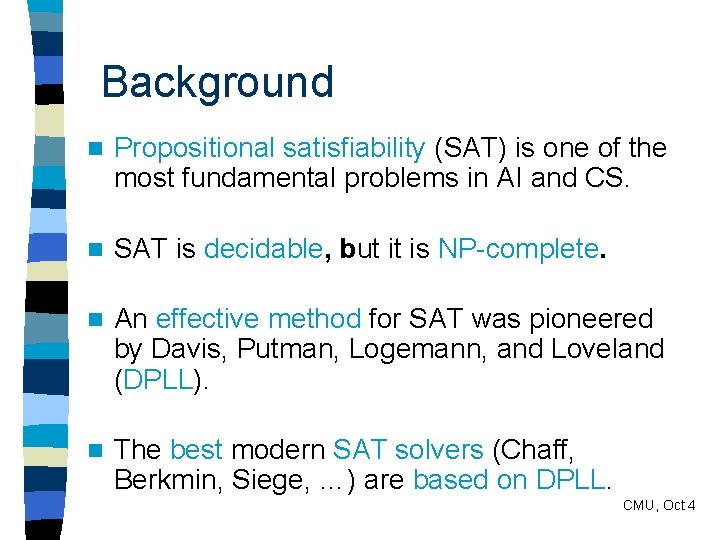Background n Propositional satisfiability (SAT) is one of the most fundamental problems in AI and CS. n SAT is decidable, but it is NP-complete. n An effective method for SAT was pioneered by Davis, Putman, Logemann, and Loveland (DPLL). n The best modern SAT solvers (Chaff, Berkmin, Siege, …) are based on DPLL. CMU, Oct 4The DPLL Procedure as a Calculus Context (partial assignment) CMU, Oct 4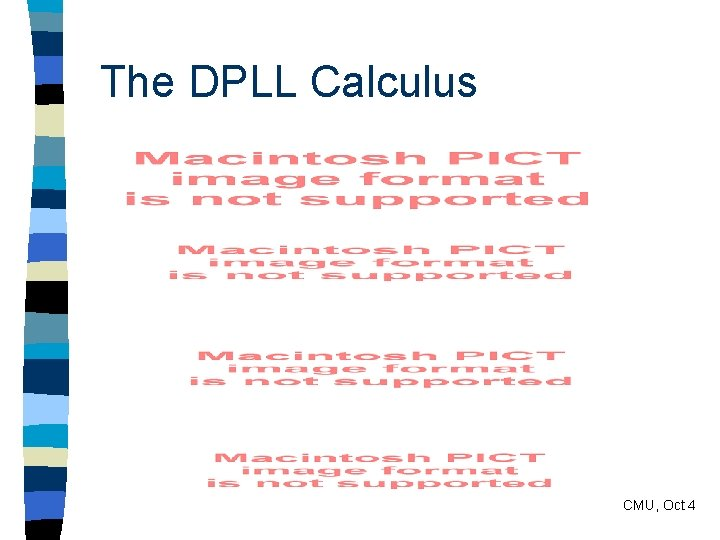The DPLL Calculus CMU, Oct 4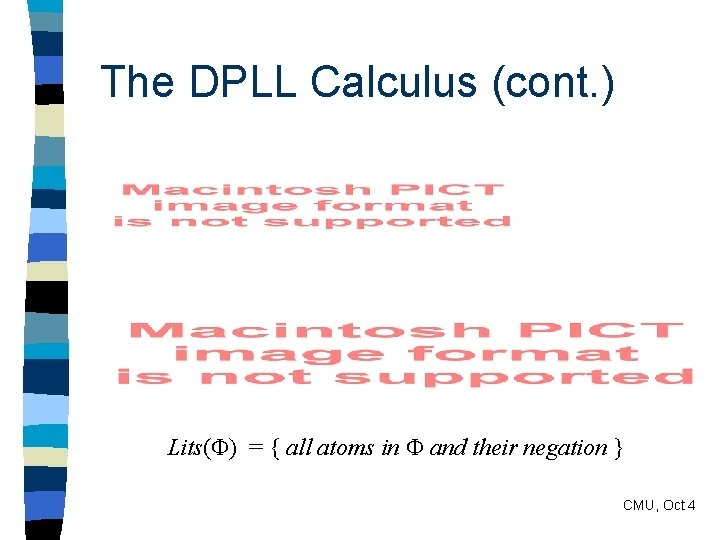The DPLL Calculus (cont. ) Lits( ) = { all atoms in and their negation } CMU, Oct 4Correctness of DPLL = { propositional clauses } Prop. Every derivation tree of |- is finite. Prop. is satisfiable iff |- has a derivation tree with a successful branch. CMU, Oct 4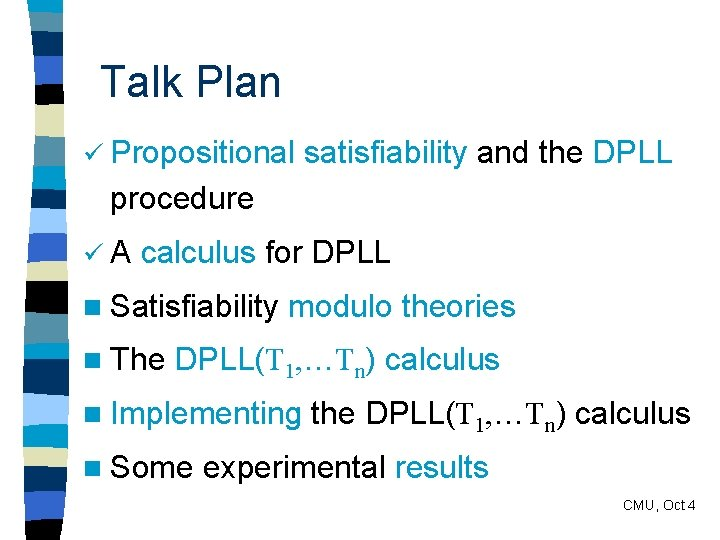Talk Plan ü Propositional satisfiability and the DPLL procedure üA calculus for DPLL n Satisfiability n The modulo theories DPLL(T 1, …Tn) calculus n Implementing n Some the DPLL(T 1, …Tn) calculus experimental results CMU, Oct 4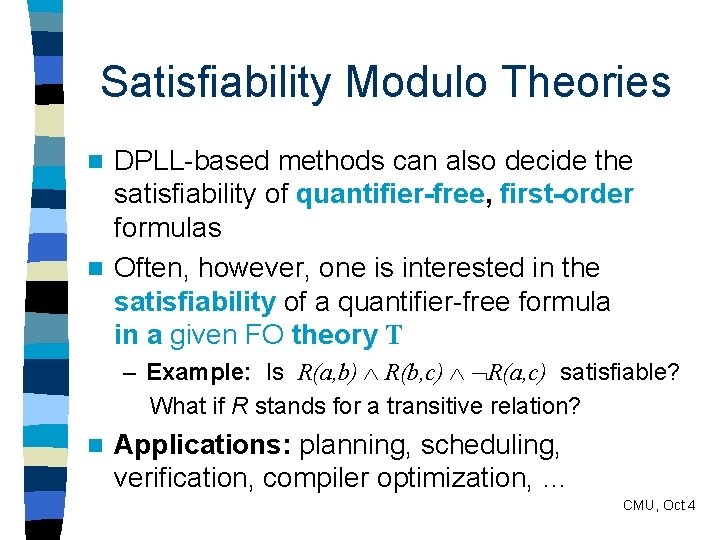Satisfiability Modulo Theories DPLL-based methods can also decide the satisfiability of quantifier-free, first-order formulas n Often, however, one is interested in the satisfiability of a quantifier-free formula in a given FO theory T n – Example: Is R(a, b) R(b, c) R(a, c) satisfiable? What if R stands for a transitive relation? n Applications: planning, scheduling, verification, compiler optimization, … CMU, Oct 4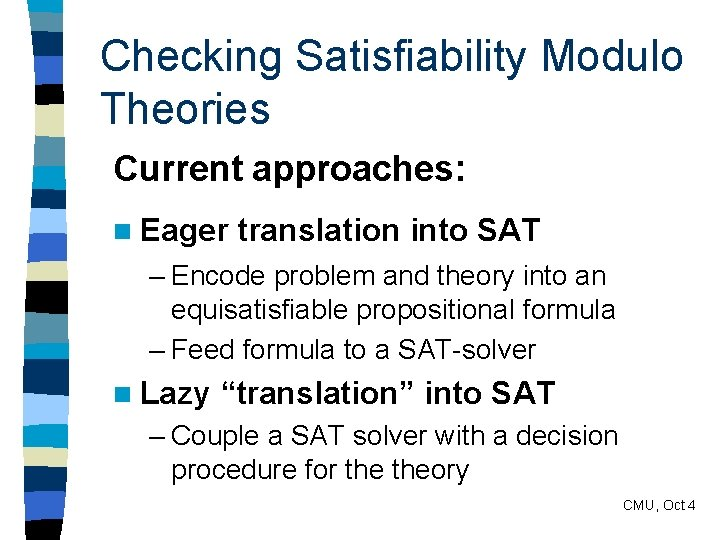Checking Satisfiability Modulo Theories Current approaches: n Eager translation into SAT – Encode problem and theory into an equisatisfiable propositional formula – Feed formula to a SAT-solver n Lazy “translation” into SAT – Couple a SAT solver with a decision procedure for theory CMU, Oct 4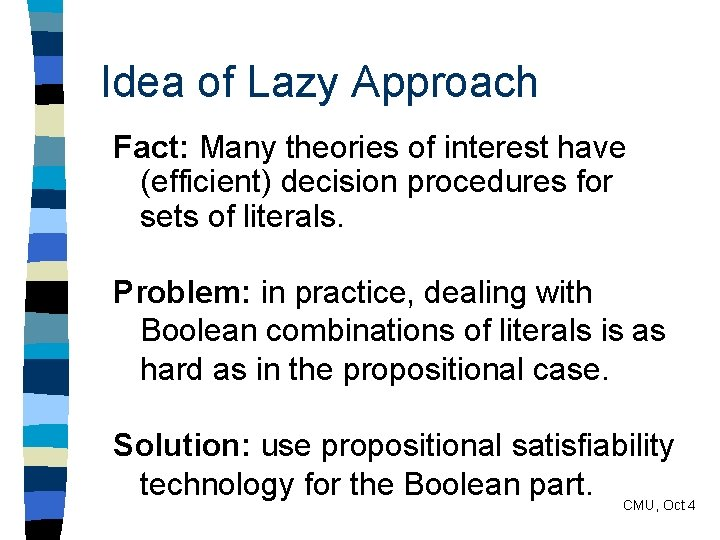Idea of Lazy Approach Fact: Many theories of interest have (efficient) decision procedures for sets of literals. Problem: in practice, dealing with Boolean combinations of literals is as hard as in the propositional case. Solution: use propositional satisfiability technology for the Boolean part. CMU, Oct 4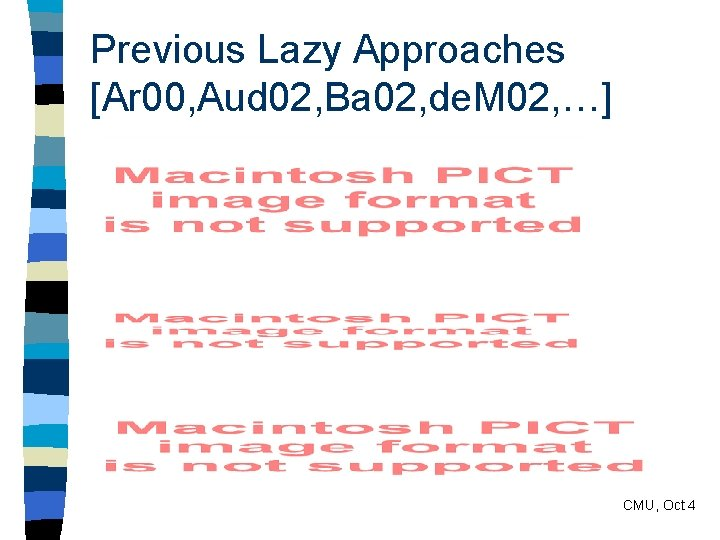Previous Lazy Approaches [Ar 00, Aud 02, Ba 02, de. M 02, …] CMU, Oct 4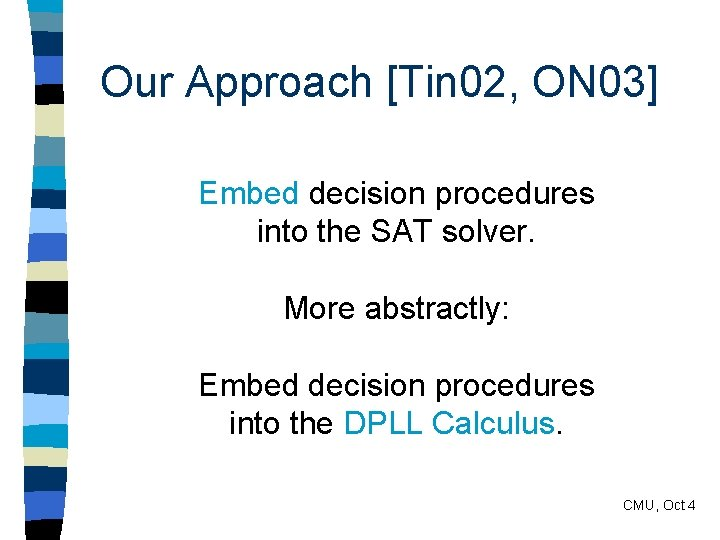Our Approach [Tin 02, ON 03] Embed decision procedures into the SAT solver. More abstractly: Embed decision procedures into the DPLL Calculus. CMU, Oct 4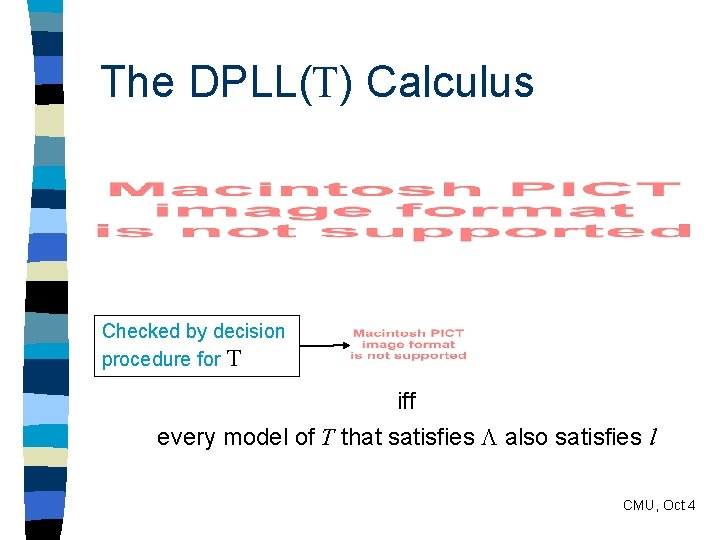The DPLL(T) Calculus Checked by decision procedure for T iff every model of T that satisfies also satisfies l CMU, Oct 4The DPLL(T) Calculus CMU, Oct 4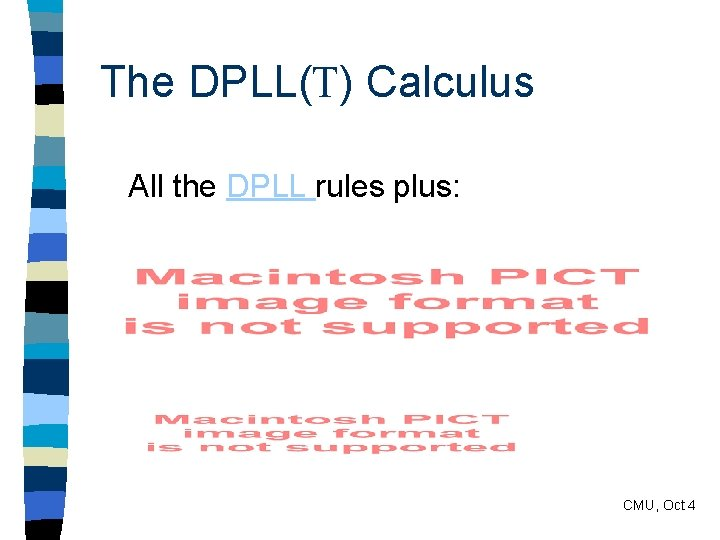The DPLL(T) Calculus All the DPLL rules plus: CMU, Oct 4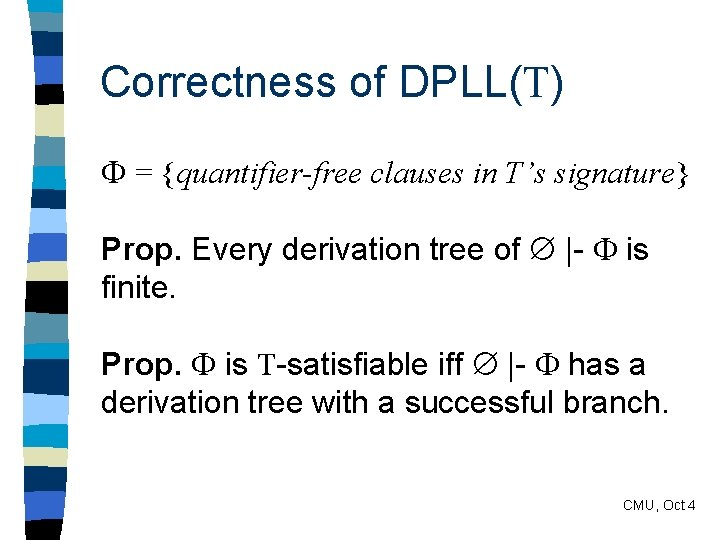Correctness of DPLL(T) = {quantifier-free clauses in T’s signature} Prop. Every derivation tree of |- is finite. Prop. is T-satisfiable iff |- has a derivation tree with a successful branch. CMU, Oct 4Satisfiability Modulo Multiple Theories n Let T 1, …, Tn be distinct theories with resp. decision procedures P 1, …, Pn n How can we reason over all of them with DPLL(T)? n Quick Solution: – Combine P 1, …, Pn with the Nelson-Oppen method into a decision procedure for T 1 … Tn – Use DPLL(T) with T = T 1 … Tn CMU, Oct 4Satisfiability Modulo Multiple Theories n Let T 1, …, Tn be distinct theories with resp. decision procedures P 1, …, Pn n How can we reason over all of them with DPLL(T)? n Better Solution: – Embed the Nelson-Oppen method directly into the calculus – Turn DPLL(T) into DPLL(T 1, …, Tn ) CMU, Oct 4The DPLL(T 1, …, Tn) Calculus: Preliminaries n Let n=2, for simplicity n Let Ti be a theory of signature i for i=1, 2, with 1 2 = n Assume wlog that each input literal has signature 1 or 2 (no mixed literals) CMU, Oct 4The DPLL(T 1, …, Tn) Calculus: Notation n Lits( , i) = { i-atoms in and their negation} n i = { i-literals of } n s = { x = y | x, y vars( 1) vars( 2) } CMU, Oct 4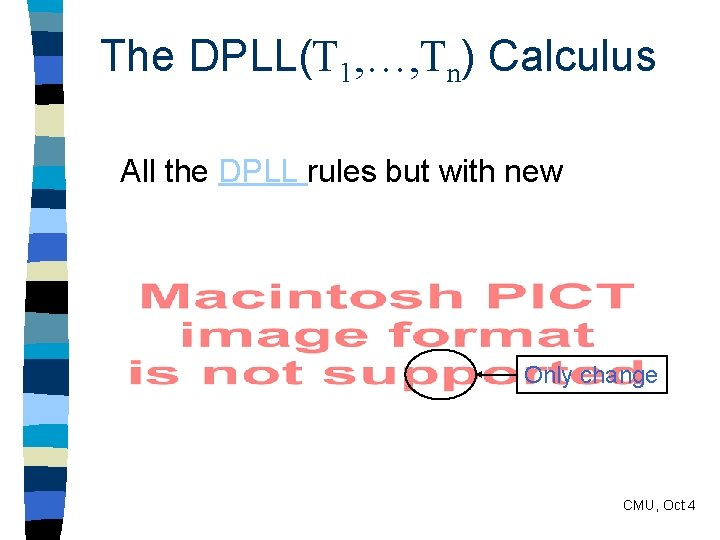The DPLL(T 1, …, Tn) Calculus All the DPLL rules but with new Only change CMU, Oct 4The DPLL(T 1, …, Tn) Calculus (cont. ) New theory rules (i=1, 2): CMU, Oct 4Correctness of DPLL(T 1, …, Tn) A theory T is stably infinite iff = {T-satisfiable quantifier-free in every qff isclauses satisfiable (T 1. . . T in an infinite modeln)’s of Tsignature } Prop. If T 1, …, Tn are • stably-infinite and • pairwise signature-disjoint then is (T 1. . . Tn)-satisfiable iff |- has a derivation tree with a successful branch. CMU, Oct 4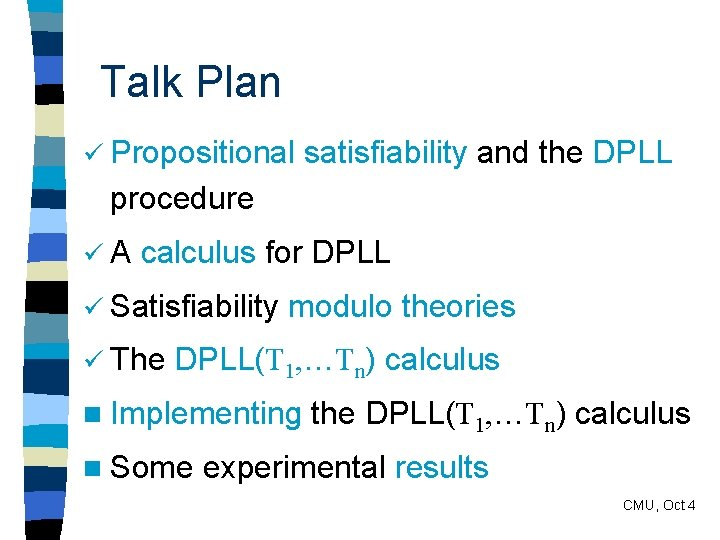Talk Plan ü Propositional satisfiability and the DPLL procedure üA calculus for DPLL ü Satisfiability ü The modulo theories DPLL(T 1, …Tn) calculus n Implementing n Some the DPLL(T 1, …Tn) calculus experimental results CMU, Oct 4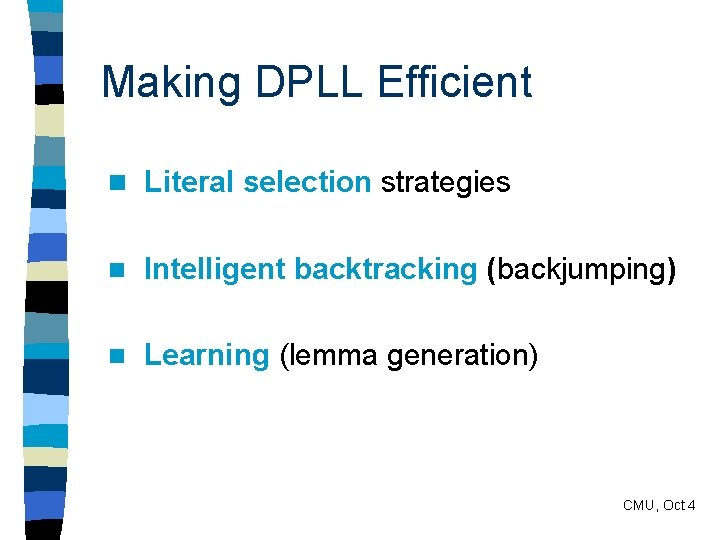Making DPLL Efficient n Literal selection strategies n Intelligent backtracking (backjumping) n Learning (lemma generation) CMU, Oct 4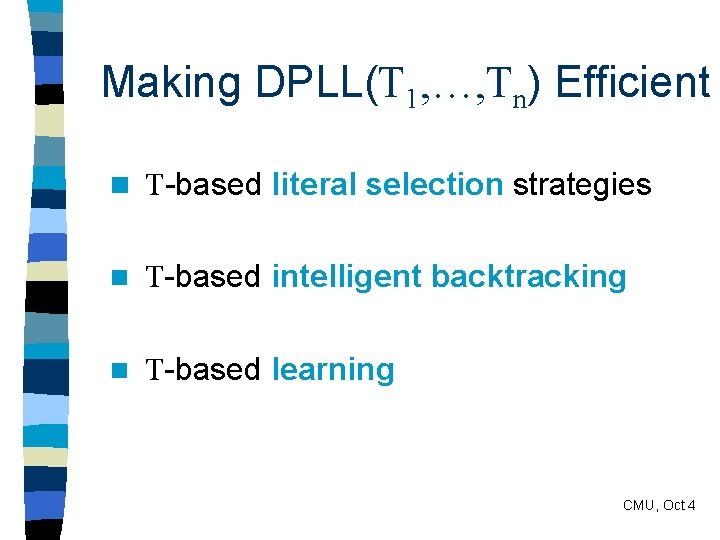Making DPLL(T 1, …, Tn) Efficient n T-based literal selection strategies n T-based intelligent backtracking n T-based learning CMU, Oct 4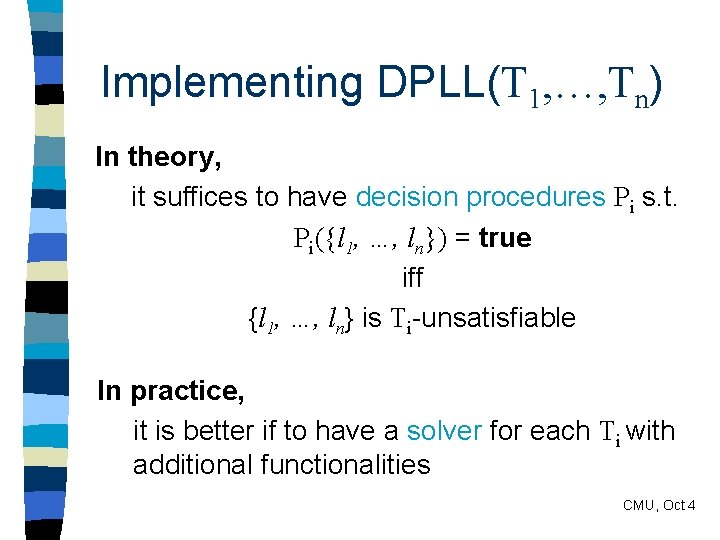Implementing DPLL(T 1, …, Tn) In theory, it suffices to have decision procedures Pi s. t. Pi({l 1, …, ln}) = true iff {l 1, …, ln} is Ti-unsatisfiable In practice, it is better if to have a solver for each Ti with additional functionalities CMU, Oct 4Ti-solver Interface type status = Valid | Unsat | Undef type lit = sign * pred Class Solver { attr context : lit list meth status : pred status explanation : pred lit set assert : lit set backtrack : int unit } CMU, Oct 4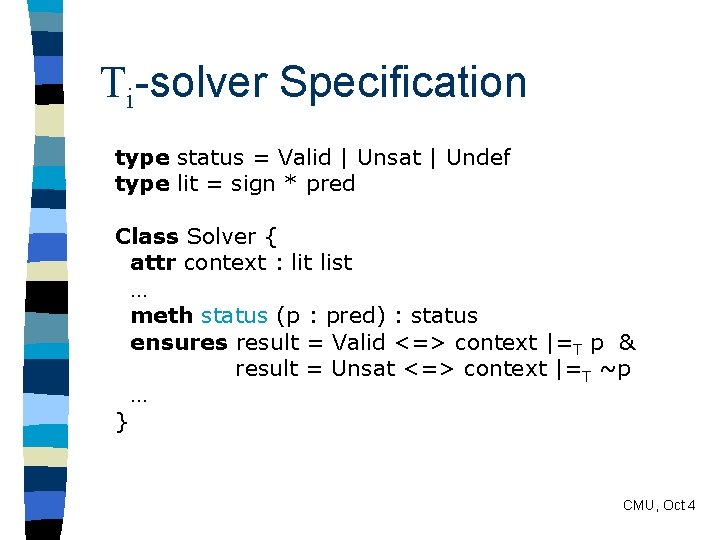Ti-solver Specification type status = Valid | Unsat | Undef type lit = sign * pred Class Solver { attr context : lit list … meth status (p : pred) : status ensures result = Valid <=> context |=T p & result = Unsat <=> context |=T ~p … } CMU, Oct 4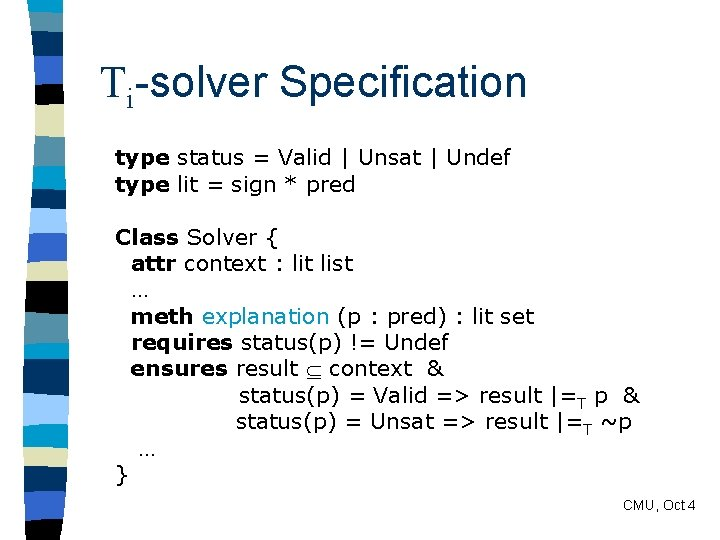Ti-solver Specification type status = Valid | Unsat | Undef type lit = sign * pred Class Solver { attr context : lit list … meth explanation (p : pred) : lit set requires status(p) != Undef ensures result context & status(p) = Valid => result |=T p & status(p) = Unsat => result |=T ~p … } CMU, Oct 4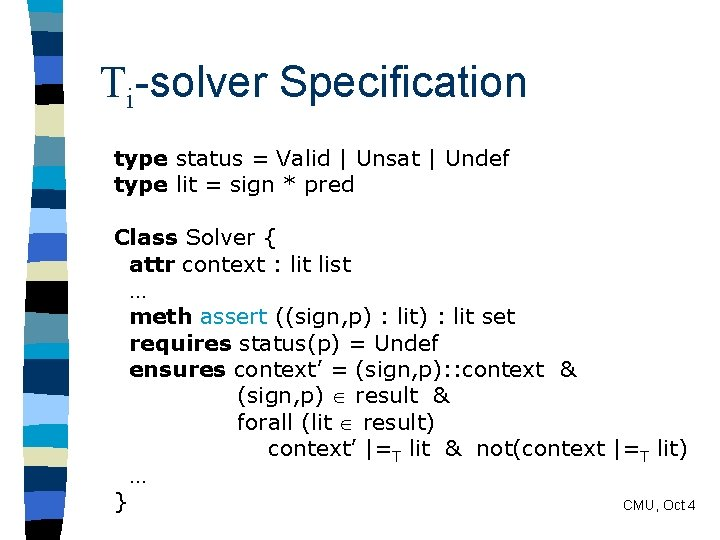Ti-solver Specification type status = Valid | Unsat | Undef type lit = sign * pred Class Solver { attr context : lit list … meth assert ((sign, p) : lit set requires status(p) = Undef ensures context’ = (sign, p): : context & (sign, p) result & forall (lit result) context’ |=T lit & not(context |=T lit) … } CMU, Oct 4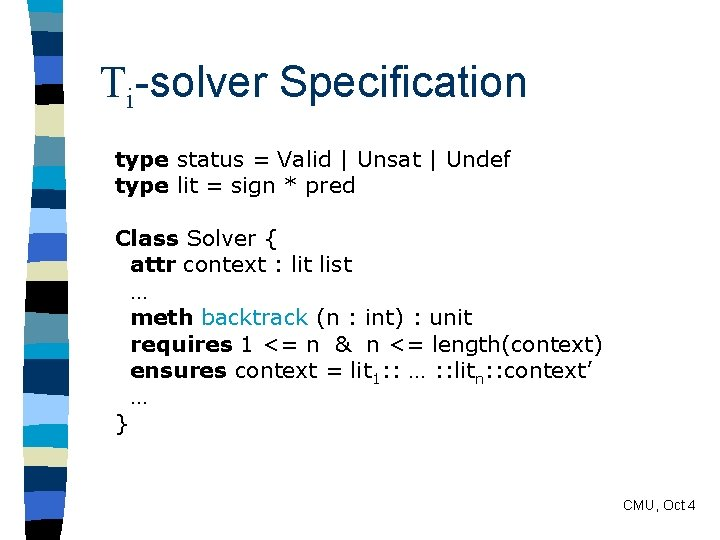Ti-solver Specification type status = Valid | Unsat | Undef type lit = sign * pred Class Solver { attr context : lit list … meth backtrack (n : int) : unit requires 1 <= n & n <= length(context) ensures context = lit 1: : … : : litn: : context’ … } CMU, Oct 4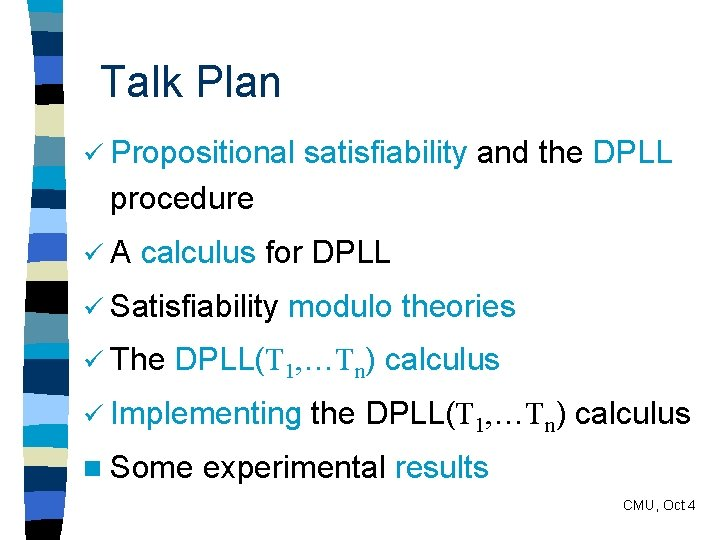Talk Plan ü Propositional satisfiability and the DPLL procedure üA calculus for DPLL ü Satisfiability ü The modulo theories DPLL(T 1, …Tn) calculus ü Implementing n Some the DPLL(T 1, …Tn) calculus experimental results CMU, Oct 4Experimental Results T = theory of successor and predecessor + free symbols p(s(x)) = x s(p(x)) = x p(x) = p(y) => x = y s(x) = s(y) => x = y 0 pn(x) 0 sn(x) pn(x) pm(x) sn(x) sm(x) (for each n > 0) (for each m, n with m > n > 0) Popular in hardware verification CMU, Oct 4Experimental Results n T-solver based on a novel congruence closure algorithm [ON 03] n DPLL(T) engine with: – Chaff-style literal selection function – 2 -watched literal unit propagation – conflict set-based backjumping – 1 st-UIP lemma generation n C implementation CMU, Oct 4Experimental Results n Benchmarks produced with UCLID tool [BLJ 02] n Comparisons with – SVC – 4 SAT-translation methods by Bryant et al. n Machine: Pentium IV, 2. 63 GHz, 512 MB RAM n Times in seconds, with timeout at 6000 s CMU, Oct 4See external table CMU, Oct 4Conclusions n DPLL(T 1, …, Tn) is a sound and complete calculus for satisfiability modulo T 1 … Tn. n General framework for integrating decision procedures into the DPLL method. n Decision procedures drive the derivation instead of just validating solutions. n Major optimization techniques from SAT can be lifted to DPLL(T 1, …, Tn). n Initial experimental results are very promising CMU, Oct 4Further Work (in Progress) n New version of the DPLL(T) calculus, to model more accurately modern SAT engines n Non-clausal version of DPLL(T) n Implementation with more theories n A DPLL(T)-based system for solving integer linear programming (optimization) problems CMU, Oct 4